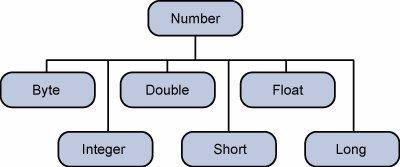# Datatypes in Java.

## Datatypes in detail:-

In this topic of Datatypes in Java we could understand about types of predefined data available in Java, Based on the data type of a variable, the operating system allocates memory and decides what can be stored in the reserved memory. Therefore, by assigning different data types to variables, you can store integers, decimals, or characters in these variables.

### There are two data types available in Java.

• Primitive Data Types.
• Reference/Object Data Types.
• Primitive Data Types

#### There are eight primitive data types supported by Java. Primitive data types are predefined by the language and named by a keyword. Let us now look into the eight primitive data types in detail.## byte

• A Byte data type is an 8-bit signed two’s complement integer.
• Minimum value is -128 (-2^7).
• Maximum value is 127 (inclusive)(2^7 -1).
• Default value is 0
• Byte data type is used to save space in large arrays, mainly in place of integers, since a byte is four times smaller than an integer.
Example: byte a = 100, byte b = -50

## short

• A short data type is a 16-bit signed two’s complement integer.
• Minimum value is -32,768 (-2^15)
• Maximum value is 32,767 (inclusive) (2^15 -1)
• Short data type can also be used to save memory as byte data type. A short is 2 times smaller than an integer
Default value is 0.
Example: short s = 10000, short r = -20000

## int

• int data type is a 32-bit signed two’s complement integer.
• Minimum value is – 2,147,483,648 (-2^31)
• Maximum value is 2,147,483,647(inclusive) (2^31 -1)
• Integer is generally used as the default data type for integral values unless there is a concern about memory.
The default value is 0 Example: int a = 100000, int b = -200000

## long

• Long data type is a 64-bit signed two’s complement integer
• Minimum value is -9,223,372,036,854,775,808(-2^63)
• Maximum value is 9,223,372,036,854,775,807 (inclusive)(2^63 -1)
• This type is used when a wider range than int is needed Default value is 0L Example: long a = 100000L,
long b = -200000L

## Float

• The float data type is a single-precision 32-bit IEEE 754 floating point
• Float is mainly used to save memory in large arrays of floating-point numbers Default value is 0.0f
• A float data type is never used for precise values such as currency Example: float f1 = 234.5f

## double

• double data type is a double-precision 64-bit IEEE 754 floating point
• This data type is generally used as the default data type for decimal values, generally the default choice
Double data type should never be used for precise values such as currency
• Default value is 0.0d Example: double d1 = 123.4

## boolean

• boolean data type represents one bit of information
• There are only two possible values: true and false
• This data type is used for simple flags that track true/false conditions
• Default value is false Example: boolean one = true

## char

• char data type is a single 16-bit Unicode character
• Minimum value is ‘\u0000’ (or 0)
• Maximum value is ‘\uffff’ (or 65,535 inclusive)
• Char data type is used to store any character Example: char letterA = ‘A’

## Java Literals

• A literal is a source code representation of a fixed value. They are represented directly in the code without any computation.
• Literals can be assigned to any primitive type variable. For example −
Byte a=68;
Char a=‘A’;
• byte, int, long, and short can be expressed in decimal(base 10), hexadecimal(base 16) or octal(base 8) number systems as well.
Prefix 0 is used to indicate octal, and prefix 0x indicates hexadecimal when using these number systems for literals. For example −
int decimal=40;
int octal=0155
int hexa=0x32